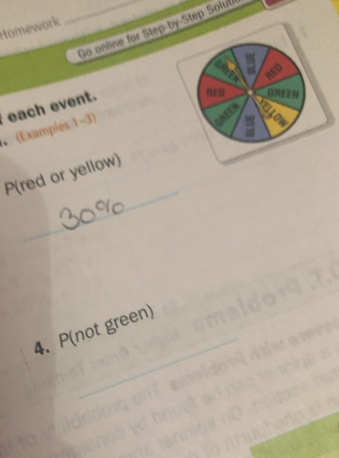#### Didn’t find what you are looking for?

Question# [Graph]P(red or yellow) 30%P(not green)

Probability
ANSWEREDP(red or yellow) 30%
P(not green)2021-03-06
Red or yellow
2 of the 8 regions are red.
The probability is the number of favorable outcomes divided by the number of possible outcomes.
P(red)=# of favorable outcomes/# of possible outcomes=$$\displaystyle\frac{{2}}{{8}}$$
1 of the 8 regions are yellow.
P(yellow)=# of favorable outcomes/# of possible outcomes=$$\displaystyle\frac{{1}}{{8}}$$
Two events are disjoint or mutually exclusive, if the events cannot occtr at the same time.
Since none of the regions are both red and yellow, the events are mutually exclusive and thus it is appropriate to use the addition rule for mutually exclusive events.
Use the Addition rule fordisjoint or mutually exclusive events: $$\displaystyle{P}{\left({A}{U}{B}\right)}={P}{\left({A}\right)}+{P}{\left({B}\right)}$$
P(red or yellow) = P(red)+P(yellow) $$\displaystyle={\left(\frac{{2}}{{8}}\right)}+{\left(\frac{{1}}{{8}}\right)}=\frac{{{2}+{1}}}{{8}}=\frac{{3}}{{8}}={0.375}={37.5}\%$$
Not green
3 of the 8 regions are green
The probability is the number of favorable outcomes divided by the number of possible outcomes.
P(green)=# of favorable outcomes/# of possible outcomes=3/8
The sum of the probability of an event and the probability of the complementary event needs to be equal 1 (P(E)+P(E)=1), which implies that the probability of the complementary event is 1 decreased by the probability of the event.
P(not green)=1-P(green)=$$\displaystyle{1}-{\left(\frac{{3}}{{8}}\right)}=\frac{{5}}{{8}}={0.625}={62.5}\%$$